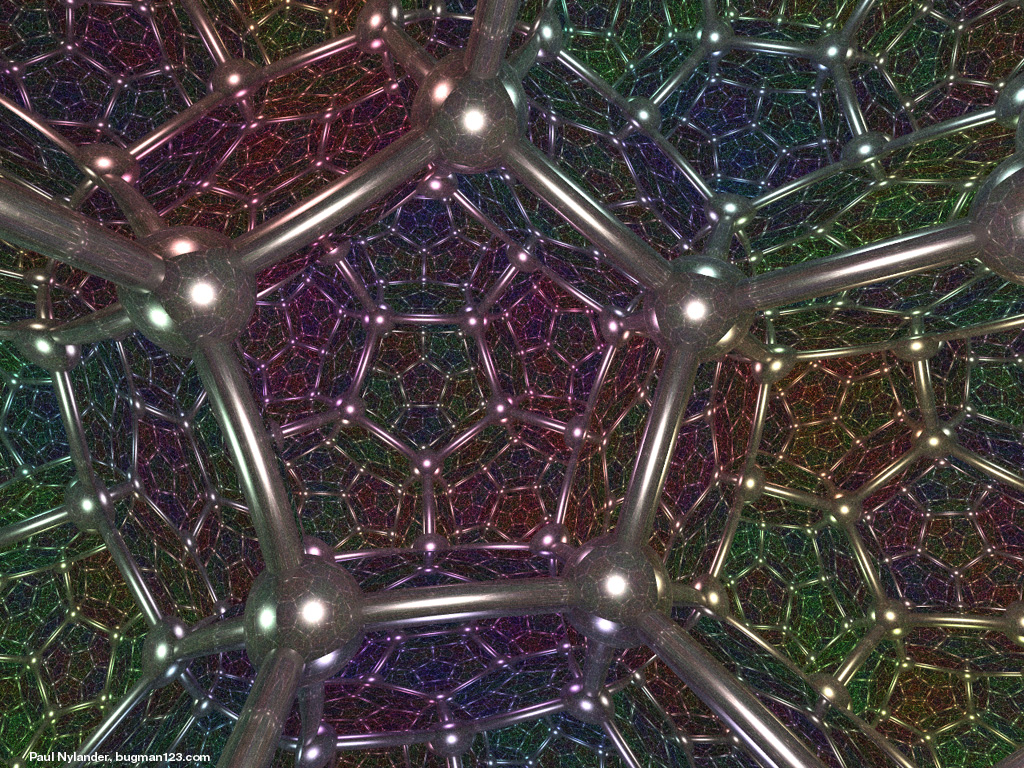### Mapping the Brain

• Spherical, Euclidean and Hyperbolic Geometries in Mapping the Brain All those folds and fissures make life difficult for a neuroscientist: they bury two thirds of the brain's surface, or cortex, where most of the information processing takes place. With so much of the brain hidden, researchers have a hard time seeing exactly which parts of the cortex are doing what and how they are related to one another. A Mercator-like flat map of the brain can be viewed in three ways:
• Euclidean, which is flat like a road map. Distance is measured or scaled as expected.
• Hyperbolic, which is disk-shaped and allows the map focus to be changed so that the chosen center is in sharp focus and the edges distorted, much like moving a magnifying glass over a piece of paper.
• Spherical, which wraps a flattened brain image around a sphere.

### Mapping the Internet### Mapping the Universe

The shape of space is directly related to whether the space is Euclidean, spherical or hyperbolic. Mathematicians are working with astronomers and physicists in order to try to solve this problem. Greek mathematicians were able to determine that the earth was round without ever leaving it. We hope to answer the most basic question about our universe in a similar manner.
Math Awareness Month
Worksheet

### A Spherical, Hyperbolic or Euclidean Universe?

Some cosmologists expect the universe to be finite, curving back around on itself. Historically, the idea of a finite universe ran into its own obstacle: the apparent need for an edge. Aristotle argued that the universe is finite on the grounds that a boundary was necessary to fix an absolute reference frame, which was important to his worldview. But his critics wondered what happened at the edge. Every edge has another side. So why not redefine the "universe" to include that other side? German mathematician Georg Riemann solved the riddle in the mid-19th century. As a model for the cosmos, he proposed the hypersphere--the three-dimensional surface of a four-dimensional ball, just as an ordinary sphere is the two-dimensional surface of a three-dimensional ball. It was the first example of a space that is finite yet has no problematic boundary. One might still ask what is outside the universe. But this question supposes that the ultimate physical reality must be a Euclidean space of some dimension. That is, it presumes that if space is a hypersphere, then that hypersphere must sit in a four-dimensional Euclidean space, allowing us to view it from the outside. Nature, however, need not cling to this notion. It would be perfectly acceptable for the universe to be a hypersphere and not be embedded in any higher-dimensional space. Such an object may be difficult to visualize, because we are used to viewing shapes from the outside. But there need not be an "outside." In fact, Einstein's Theory of Relativity is based on Riemann's model.

### Geometry of Celestial Mechanics

Mercury's orbit about the Sun is slightly more accurately predicted when Hyperbolic Geometry is used in place of Euclidean Geometry.

### Geometry of the Universe Experiments

Geometry of the Universe slides

### A Dodecahedral Universe?By gluing together corresponding sides of this 18 sided figure (for example, the pentagon faces get glued together), we obtain a hyperbolic universe. This example was discovered by Jeff Weeks.### Dodecahedron Tiling/Gluing

Notice that if you want to glue opposite faces of flat dodecahedral dice together, we will need to do so with a twist, since the straight across gluing won't work. Try to fit the flat dodecahedons together to tile our space.### In Nature